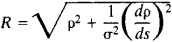# Osculating Sphere

## osculating sphere

[¦äs·kyə‚lād·iŋ ′sfir]
(mathematics)
For a curve C at a point p, the limiting sphere obtained by taking the sphere that passes through p and three other points on C and then letting these three points approach p independently along C.

## Osculating Sphere

The osculating sphere of a space curve l at a point M is the sphere having contact of order n ≥ 3 with l at M. The osculating sphere can also be defined as the limit of a variable sphere passing through four points of l as the points tend to M.

The radius of the osculating sphere iswhere ρ is the radius of curvature of l at M, ̓ is the torsion of l, and ds is the differential of arc of l.

### REFERENCE

Rashevskii, P. K. Kurs differentsial’noi geomelrii, 4th ed. Moscow, 1956.
Mentioned in ?
References in periodicals archive ?
If its pseudo-Galilean trihedron is {t(x), n(x), b(x)}, then the center of osculating sphere of r at the point r(x) is given by
If its pseudo-Galilean trihedron {t(x), n(x), b(x)}; then the center of osculating sphere of r at the point r(x) is given by

Site: Follow: Share:
Open / Close